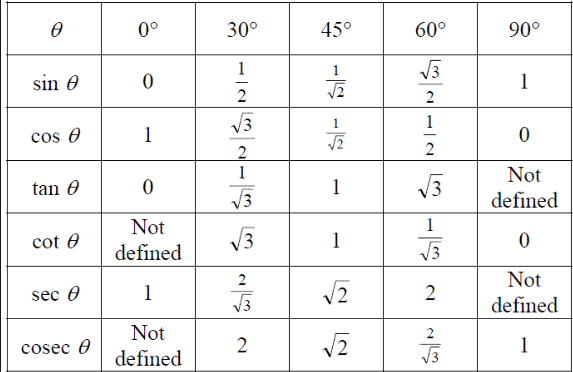Q

# I need help with - Trigonometry - JEE Main-6

1. If A, B, A – B and A + B are positive acute angles, find the values of A and B from

the equations sin (A – B) =, cos (A + B) =.

• Option 1)

A = 600 and B = 300

• Option 2)

A = 150 and B = 450.

• Option 3)

A = 450 and B = 150

• Option 4)

A = 300 and B = 300

121 Views

As we learnt

Trigonometric Ratios of Special Angles -- wherein

These are the values of trigonometric ratios for standard angles.

The equations are sin (A – B) =  = sin 300 Þ A – B = 300.         …(1)

And cos (A + B) =  = cos 600 Þ A + B = 600.                          ...(2)

From (1) and (2), A = 450 and B = 150.

Option 1)

A = 600 and B = 300

Option 2)

A = 150 and B = 450.

Option 3)

A = 450 and B = 150

Option 4)

A = 300 and B = 300

Exams
Articles
Questions